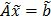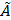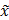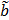Archive
Special Issues
Algebraic Modification of Trapezoidal Fuzzy Numbers to Complete Fully Fuzzy Linear Equations System Using Gauss-Jacobi Method
International Journal of Management and Fuzzy Systems
Volume 5, Issue 2, June 2019, Pages: 40-46
Received: Jul. 20, 2019; Accepted: Aug. 19, 2019; Published: Sep. 2, 2019
Authors
Ahmad Syaiful Abidin, Department of Mathematics, University of Riau, Pekanbaru, Indonesia
Sri Gemawati, Department of Mathematics, University of Riau, Pekanbaru, Indonesia
Article ToolsAbstractPDF (456KB)
Abstract
This paper will discuss algebraic modification of trapezoidal fuzzy numbers with a general form of fully fuzzy numbers iswithis n × n fuzzy matrix,fuzzy vector, andunknown fuzzy vector. The concept used in this paper is define positive or negative fuzzy numbers determined by the area on the left side of the x-axis and the right side of the x-axis. Furthermore, the concept will be applied to the multiplication of two fully fuzzy trapezoidal numbers to produce a new algebra that can be applied to a system of linear equations. At the end, an example of multiplication two fully fuzzy trapezoidal numbers using the Gauss-Jacobi method will be given. As a result compatible number will be obtained.
Keywords
Fully Fuzzy Number, Trapezoidal, New Algebra, Gauss-Jacobi Method
Ahmad Syaiful Abidin, Mashadi Mashadi, Sri Gemawati, Algebraic Modification of Trapezoidal Fuzzy Numbers to Complete Fully Fuzzy Linear Equations System Using Gauss-Jacobi Method, International Journal of Management and Fuzzy Systems. Vol. 5, No. 2, 2019, pp. 40-46. doi: 10.11648/j.ijmfs.20190502.12
References

T. Allahviranloo, S. Salahshour, M. Homayoun-Nejad dan D. Beleanu, General solution of fully fuzzy systems, Abstract and Applied Analysis, 2013, 2013, 1-9.

A. jafarian, New decomposition method for solving dual fully fuzzy linear system, Imt. Journal Fuzzy Computation and Modelling, 2, 2016, 76-85.

M. Deghan dan B. Hashemi, Iterative solution of fuzzy linear system, Applied Mathematics and Computation, 175, 2006, 645-674.

S. Gemawati, Nasﬁanti, Mashadi dan A Hadi, A new for dual fully fuzzy linear system with trapezoidal fuzzy number by QR decomposition, Journal of Physics, 1116, 2018, 1-5.

G. Malkawi, N. Ahmad dan H. Ibrahim, Solving fully fuzzy linear sys tem with the necessary an suficient to have a positive solution, Applied Mathematics and Information Sciences, 8, 2014, 1003-1019.

A. Hadi, Mashadi dan S. Gemawati, On fuzzy n-inner product spaces, Journal of Physics, 020010, 2017, 1-6.

S. I. Marni, Mashadi, S. Gemawati, Solving dual fully fuzzy linear system by use factorizations of the coeficient matrix for trapezoidal fuzzy number, Bulletin of Mathematics, 2, 2018, 145-156.

N. J. Karthik dan E. Chandrasekaran, Solving fully fuzzy linear system with trapezoidal fuzzy number matrices by partitioning, International Jo- urnal of Computer Applications, 64, 2013, 35-38.

A. Kumar, Neetu, dan A. Bansal, A new method to solve fully fuzzy linear system with trapezoidal fuzzy number, Canadian Journal on Science and Engineering Mathematics, 1, 2010, 45-56.

A. Kumar, J. Kaur dan P singh, A new method for solving fuzzy linier programs with trapezoidal fuzzy numbers, Journal of Fuzzy, 2011, 2011, 1-12.

A. Kumar and J. Kaur, Comentary on “Calculating fuzzy inverse matrix using fuzzy linear equation system”, Applied Soft Computing, 58, 2017, 324-327.

L. A. Zadeh, Fuzzy Sets, Information and Control, 8, 1965, 338-353.

L. Abdullah dan N. A. Rahman, Jacobi-based methods in solving fuzzy linier system, International Journal of Mathematical and Computational Science, 7, 2013, 402-408.

Mashadi, A new method for dual fully fuzzy linier system by use LU factorizations of the coeficient matrix, Jurnal Matematika dan Sains, 15, 2010, 101-106.

S. Moloudzadeh, P. Darabi dan H. Khandani, The pseudo invers matrices to solve general fully fuzzy linear system, Journal of Soft Computing and Applications, 2013, 2013, 1-11.

S. H. Nasheri dan M. Gholami, Linear system of equation with trapezoidal fuzzy numbers, The Journal of Mathematics and Computer Science, 3, 2011, 71-79.

S. Das dan S. Chakraverty, Numerical solution of fuzzy system of linear equation, Applications and Applied Mathematics, 7, 2012, 334-356.
PUBLICATION SERVICES